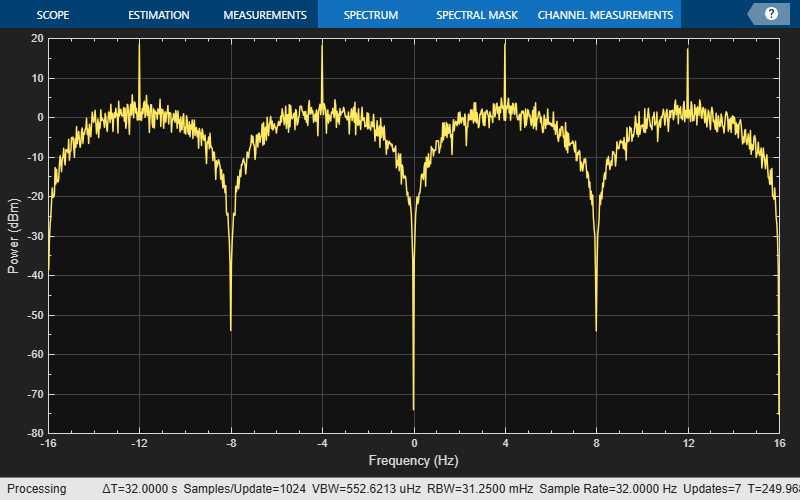Documentation

# fskmod

Frequency shift keying modulation

## Syntax

```y = fskmod(x,M,freq_sep,nsamp) y = fskmod(x,M,freq_sep,nsamp,Fs) y = fskmod(x,M,freq_sep,nsamp,Fs,phase_cont) y = FSKMOD(x,M,freq_sep,nsamp,Fs,phase_cont,symbol_order) ```

## Description

`y = fskmod(x,M,freq_sep,nsamp)` outputs the complex envelope `y` of the modulation of the message signal `x` using frequency shift keying modulation. `M` is the alphabet size and must be an integer power of 2. The message signal must consist of integers between 0 and `M-1`. `freq_sep` is the desired separation between successive frequencies in Hz. `nsamp` denotes the number of samples per symbol in `y` and must be a positive integer greater than 1. The sampling rate of `y` is 1 Hz. By the Nyquist sampling theorem, `freq_sep` and `M` must satisfy `(M-1)*freq_sep <= 1`. If `x` is a matrix with multiple rows and columns, the function processes the columns independently.

`y = fskmod(x,M,freq_sep,nsamp,Fs)` specifies the sampling rate of `y` in Hz. Because the Nyquist sampling theorem implies that the maximum frequency must be no larger than `Fs/2`, the inputs must satisfy ```(M-1)*freq_sep <= Fs```.

`y = fskmod(x,M,freq_sep,nsamp,Fs,phase_cont)` specifies the phase continuity. Set `phase_cont` to `'cont'` to force phase continuity across symbol boundaries in `y`, or `'discont'` to avoid forcing phase continuity. The default is `'cont'`.

`y = FSKMOD(x,M,freq_sep,nsamp,Fs,phase_cont,symbol_order)` specifies how the function assigns binary words to corresponding integers. If `symbol_order` is set to `'bin'` (default), the function uses a natural binary-coded ordering. If `symbol_order` is set to `'gray'`, it uses a Gray-coded ordering.

## Examples

collapse all

Generate an FSK modulated signal and display its spectral characteristics.

Set the function parameters.

```M = 4; % Modulation order freqsep = 8; % Frequency separation (Hz) nsamp = 8; % Number of samples per symbol Fs = 32; % Sample rate (Hz) ```

Generate random M-ary symbols.

```x = randi([0 M-1],1000,1); ```

Apply FSK modulation.

```y = fskmod(x,M,freqsep,nsamp,Fs); ```

Create a spectrum analyzer System object? and use its `step` method to display a plot of the signal spectrum.

```h = dsp.SpectrumAnalyzer('SampleRate',Fs); step(h,y) ```Sklar, Bernard. Digital Communications: Fundamentals and Applications. Upper Saddle River, NJ: Prentice-Hall, 2001.